V1

2023/05/04阅读：8主题：萌绿

# Typescript学习(第一弹)

## Typescript的基础类型

### Boolean类型

``let bool: boolean = true;``

### Number类型

``let num: number = 222;``

### String类型

``let str: string = '码上coding';``

### 数组Array类型

• 对数组类型的定义有两种方式:
``let arr1: number[] = [1,2,3];let arr2: Array<number | string> = [1,2,'码上coding']; // Array是ts预定义的泛型接口``

### 元组tuple类型

• 表示一个已知数量和类型的数组,可以理解为他是一种特殊的数组
``// 限制了数组元素的个数和类型，let tuple: [number, string] = [0, '1'];// tuple.push(2) 元组可以使用push方法插入新元素，是元组越界bug，// tuple 会报错，不允许访问``

### 函数

``let add = (a: number,b: number): number => a + blet plus: (a: number,b: number) => number // 函数类型plus = (a,b) => a + b``

### object对象

• object 类型用于表示所有的非原始类型，即我们不能把 number、string、boolean、symbol等 原始类型赋值给 object。在严格模式下，null 和 undefined 类型也不能赋给 object。
• 大 Object 代表所有拥有 toString、hasOwnProperty 方法的类型 所以所有原始类型、非原始类型都可以赋给 Object(严格模式下 null 和 undefined 不可以)
• {} 空对象类型和大 Object 一样 也是表示原始类型和非原始类型的集合
``let obj: object = { x:1, y:2 }obj = 1; // 报错obj = "a"; // 报错obj = true; // 报错obj = null; // 报错obj = undefined; // 报错obj = {}; // 正确let obj2: { x:number, y:number } = { x:1, y:2 }obj2.x = 3;let obj3: Object = { x:1, y:2 }obj3 = 1; // 正确obj3 = "a"; // 正确obj3 = true; // 正确obj3 = null; // 报错obj3 = undefined; // 报错obj3 = {}; // 正确let obj4: {}obj4 = 1; // 编译正确obj4 = "a"; // 编译正确obj4 = true; // 编译正确obj4 = null; // 报错obj4 = undefined; // 报错obj4 = {}; // 正确``

### Class类

``class Car {  name: string;  color: number;  constructor(name: string, color: number) {    this.name = name;    this.color = color;  }  likeColor(): void {    console.log(`I like \${this.color}`);  }}``

### Symbol

``let s1: symbol = Symbol();let s2 = Symbol();``

### undefined, null

``// undefined, null  不能赋值为其他类型let unde: undefined = undefined;let nu: null = null;// 关闭tsconfig.json里的strictNullChecks:false,其他类型的值可以赋值为undefined和 null // num = undefined// num = null``

### void类型

• void是一个操作符，可以让任何表达式返回undefined
``/** eg:void 0 因为undefined可以赋值所以 void 可以确保返回的一定是undefined*/let noReturn = ():void => {}``

### any类型

• 会跳过类型检查器对值的检查，任何值都可以赋值给any类型
``let x:any = 2x = [];x = () => {};``

### Unknown 类型

• unknown与any一样，所有类型都可以分配给unknown
``  let value: unknown = 1;  value = "qqq";  value = false;``
• unknown与any的区别
• 任何类型的值可以赋值给any，同时any类型的值也可以赋值给任何类型
• unknown 任何类型的值都可以赋值给它，但它只能赋值给unknown和any

### never类型

• 永远不会有返回值的类型
``// never,永远不会有返回值的类型let error = ():never => { // 抛出异常    throw new Error('error')}let endless = ():never => { // 死循环    while(true){}}``

### Enum枚举类型

• 一组具有名字的常量集合，定义后不能修改

#### 数字枚举

``enum Role {    Alice = 1, // 可以指定为1，不写默认是0开始递增    Jack,    Apple}console.log(Role) // 打印出来类似对象``
• 打印出来类似对象,数字和字符串都作为key,说明有反向映射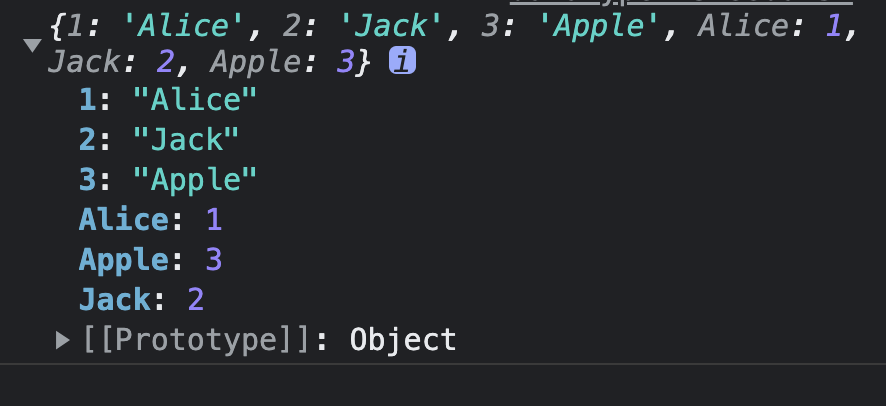#### 字符串枚举

``enum Test {    First='Hello',    Second='World'}``
• 打印出来，赋值的字符串作为了value，没有作为key,说明没有反向映射！！！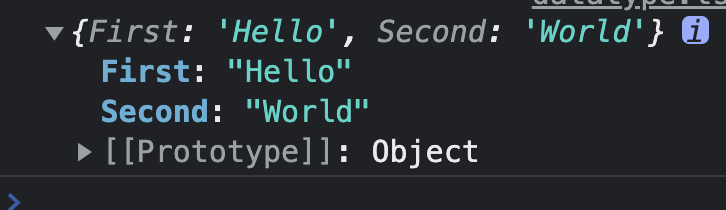#### 异构枚举

• 数字枚举和字符串枚举混用,容易混淆，不建议使用
``enum Answer {    First,    Second='ok'}``
• 只有数字枚举有反向映射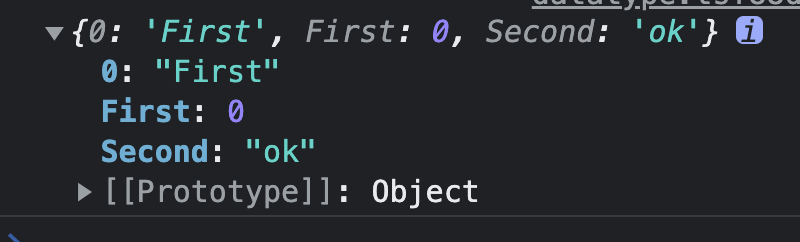#### 枚举成员

• const 常量成员
• 没有初始值
• 对已有成员的引用
• 常量的表达式，在编译的时候计算出结果，以常量的形式出现在运行时环境
• computed 需要被计算的成员
• 一定要赋值，不然会报错
• 不会在编译阶段计算，在运行时才会被计算
``enum Char {    // const 常量成员    a, // 没有初始值    b = Char.a, // 对已有成员的引用    c = 1 + 3, // 常量的表达式，在编译的时候计算出结果，以常量的形式出现在运行时环境    // computed 需要被计算的成员, 一定要赋值，不会在编译阶段计算，在运行时才会被计算    d = Math.random(),     e = 'abc'.length,}console.log(Char)``
• 编译后 const成员的值已经计算出结果了，computed成员的值被保留了，要运行时才会被计算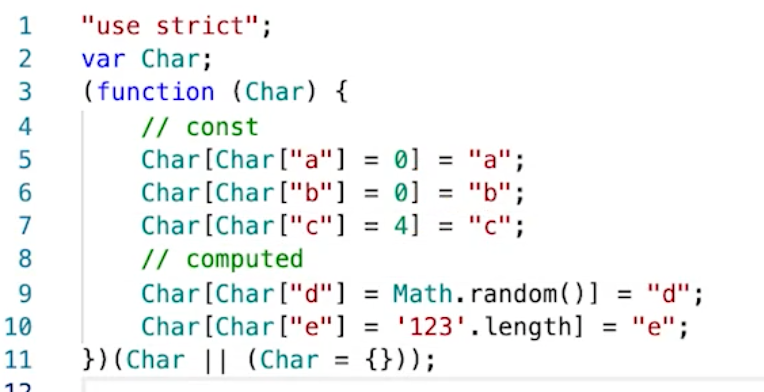#### 常量枚举

• 用const定义的枚举就是常量枚举，会在编译阶段被移除
• 当我们不需要对象，只需要对象的值的时候就可以使用常量枚举，可以减少编译环境的代码
``const enum Month {    Jan,    Feb,    Mar}let month = [Month.Jan, Month.Feb, Month.Mar]``
• 编译后，只是把枚举的值编译出来了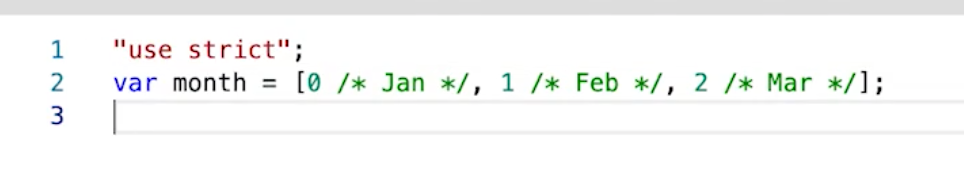#### 枚举类型

• 不同类型是不可以比较的
``enum E { a,b } // 都没有初始值enum F { a = 1,b = 2 } // 初始值都为数字enum G { a = '1',b = '2' } // 初始值都为字符串// 不同类型是不可以比较的let e:E = 3let f:F = 3// e === f  会报错let e1:E.a = 1let e2:E.b = 2let e3:E.a = 1// e1 === e2 也会报错// e1 === e3 可以let g1:G = G.blet g2:G.a = G.a``

## 关注我不迷路V1